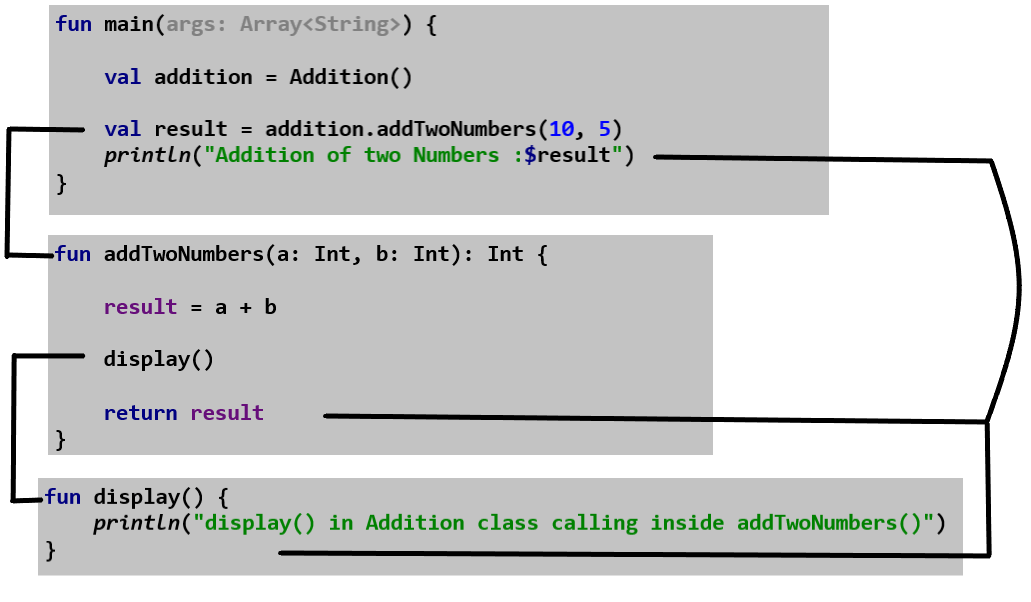# Kotlin Functions

In this article, you’ll learn about Kotlin functions, how to define a function and use them in your program with the help of examples.

### Functions

A Kotlin function is a collection of statements that are grouped together to perform an operation.

Functions allow us to reuse the code without retyping the code i.e. you can write a method once, and use it multiple times. It also makes code more readable and easier to debug.

In Kotlin, functions can be declared at the top, which means you do not need to create a class to hold a function, which you are required to do in other languages such as Java, C# or Scala.

### Types of Functions

In Kotlin, depending on whether a function is defined by the user or available in the standard library, functions are of two types:

• Standard Library Functions
• User-defined Functions

#### Standard Library Functions

Standard library functions are built-in functions in Kotlin that are already defined in standard libraries and available for use.

Examples of Standard library functions are:

• sqrt() – calculates the square root of a number.
• print() – prints the message to standard output.
• max() – returns the greater of two values.
• pow() – calculates the nth power of a number.
• compareTo() – compares two values and return boolean.
• toInt() – converts a number to integer value.
##### For example:
```fun main(args: Array<String>) {

var average = arrayOf(1, 2, 3, 4, 5, 6, 7, 8, 9, 10).average()

print("The average of N numbers is: \$average")
}```
##### Output:
`The average of N numbers is: 5.5`
##### Example explained
• arrayOf(), average() and print() are built-in functions.
• arrayOf(): require some arguments like integers, double, etc. to create an array.
• average(): used to find the average of values passed to an array.
• print(): used to print the message to standard output.

#### User-defined Functions

A function which is defined by the user is called user-defined function.

To work with user-defined function, firstly we have to define a function then call it or use it.

#### Defining a method

Before you can use a function (call a method), you need to define it.

##### Syntax:

The syntax of defining a function:

```fun functionName(Parameter list): returnType  {
//Function body
}```

More generally, function declarations have the following components, in order:

fun: keyword to define a function.

functionName: name of the function, later use to call the function.

Note: You can give any name to a function. But it’s more conventional to name it upon the task it performs. As per the naming convention, a method should start with lower case and if it contains more than one word then every word starting should be of upper case.For example: calculateAddition, displayStudentDetails etc.

Parameter list: Parameters are the values passed to a function. You can pass any no of arguments to a function.

In Kotlin, function parameters are defined like this: parameter name: type(datatype)

returnType: defines the data type of the value returned by the function or Unit (declaration is optional) if a function does not return a value.

Function body:  The code inside curly braces { } is the body of the function. It defines what the method actually does, how the parameters are manipulated with statements and what values are returned.

##### Example:
```fun max(x: Int, y: Int): Int {
//function body
if (x > y) {
println("The greater number is: \$x")
return x
} else {
println("The greater number is: \$y")
return y
}
}```
##### Example explained:
• max:  function name
• x: Int, y: Int (parameter list):  x and y are the parameters name of Int (integer) data type.
• Int (returnType): defines that max() function returns integer value.

#### Single-expression functions

In Kotlin, when a function returns a single expression, the curly braces can be omitted and the body is specified after a  =  symbol:

```fun max(x: Int, y: Int): Int = if (x > y) x else y

fun add(x: Double, y: Double): Double = x + y

fun data(x: Int): Unit = print("Value of x is \$x")```

Note: Functions with block body must always specify return types explicitly while working with single-expression functions declaring the return type explicitly is optional.

```fun max(x: Int, y: Int) = if (x > y) x else y

fun add(x: Double, y: Double) = x + y

fun data(x: Int) = print("Value of x is \$x")```

#### Calling a method

Now we have defined a method, we need to use it. For that, we have to call the method to use its functionality.

##### Syntax:

Syntax of calling  a method:

`functionName(Parameter list)`

The way of calling the max() method which we have defined above:

`max(5 , 9)`

In a program, when a compiler comes to a line containing the function call, control jumps to the definition of that function and executes all the instructions one by one.

Control is transferred back only when the function reaches closing braces or there any return statement.

##### Example:
```//defining max() function
fun max(x: Int, y: Int): Int {
if (x > y) {
println("The greater number is: \$x")
return x
} else {
println("The greater number is: \$y")
return y
}
}

fun main(args: Array<String>) {
//calling max() function
var result = max(9, 5)
println("The maximum of two numbers 5 and 9 is \$result")
}```
##### Output:
```The greater number is: 9
The maximum of two numbers 5 and 9 is 9```

### Example: Kotlin Functions

```class Addition {
var result = 0

//function with two parameters and
//returning integer value.therefore,returnType is Int
fun addTwoNumbers(a: Int, b: Int): Int {

result = a + b

//calling display() method within the same class
display()

return result
}

//display() function definition
//function with no parameter and
//returning nothing.therefore,returnType is Unit
//Unit(returnType) declaration is optional
fun display(): Unit {
}
}

fun main(args: Array<String>) {
// creating an instance of Addition class
```display() in Addition class calling inside addTwoNumbers()